## Inserting formulas

A table cell can display the result of a formula combining values of other cells with arithmetic operators and functions. Formulas are recalculated whenever values in the table change, so they’re always up to date.

Any cell starting with the character “=” is treated as a formula. To enter or edit formulas, use the Table toolbar’s edit field. Note that you can only select or edit an entire formula, not just part of it.

PagePlus lets you make use of relative or absolute cell references. The latter makes use of the \$ symbol before a column and/or row reference (\$A\$1, \$A1, A\$1) and can be used when copying and pasting formulas, in order to reference a constant value throughout your table.

• Remember to enable the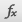Spreadsheet functions button (on the Table context toolbar) to allow you to create formulas.

#### To display a cell’s formula for editing in the Table toolbar’s edit field:

• Click to select the cell containing the formula.

#### To enter a formula:

• Click the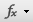Function button on the Table toolbar and choose a specific function from the drop-down menu.
For example, if you start with a blank cell and choose SUM(), PagePlus adds “=SUM()” to the edit field and positions the text cursor between the brackets so that you can type numbers or cell references straight away.
– or -

• Click the Function button once to insert an equal sign (=) into the edit field (or type “=” yourself), then continue to type the formula.

Click the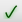Accept button to update the selected cell or click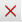Cancel.

Formulas can be made up from any of the following:

• Operators

• Percentages

• Values

• Functions

#### Operators

PagePlus allows use of the +, -, * and / arithmetic operators. The usual order of evaluation is applied, with multiplication and division being evaluated before addition and subtraction. For example:

4 + 3 * 2 = 10 not 14

Operators with equal order of evaluation are performed left to right. To override the default order, enclose portions of the formula in parentheses:

(4 + 3) * 2 = 14

For ease in entering a range of cells into a formula, PagePlus detects these special characters:

+ – * / = : , (

When editing a formula, if you place the text cursor immediately after a special character, the cursor changes and you can now click on a cell or drag a range of cells to automatically enter their references into the formula.

#### Percentages

PagePlus treats percentage calculations differently depending on the operator (+, -, * or /) used with percentage symbol (%). For example:

2000 + 10% = 2200  Adds 10% to 2000

2000 – 10% = 1800  Subtracts 10% from 2000

2000 * 10% = 200  Returns 10% of 2000

200 / 2000% = 10  Returns the percentage 200 is of 2000

#### Values

Values in formulas can be the following:

• Numbers e.g. 1, 13, -89.5

• Single cell references, e.g. A3, B6, C12. (See Cell references below.)

• Ranges of cell references. These are specified by the starting cell followed by a colon, then the end cell. For example: A1:B5 specifies every cell between A1 and B5 inclusive.

The simplest way to enter cell references or ranges of cells is to click on a cell or drag out a range. Clicking on the column or row label selects the whole column or row, respectively (e.g., C1:C15 or A9:D9).

Absolute cell references using the \$ symbol before a column and/or row reference (\$A\$1, \$A1, A\$1) can be used when copying and pasting formulas, in order to reference a constant value throughout your table.

#### Functions

PagePlus has a large number of built-in functions. To see all of them, simply click theFunction button and explore the drop-down menu (watch the Hintline for explanations).

• ABS( ) – returns the absolute value of a number

• AVG( ) – returns the arithmetic mean of its arguments

• CONCATENATE( ) – returns a concatenation of its argument into a single string

• COUNT( ) – counts how many numeric values are in its arguments

• MAX( ) - returns the maximum value from its argument

• MIN( ) - returns the minimum value from its argument

• PI( ) - calculates the value of Pi

• POW( ) - returns a number to the power of its argument

• ROUND( ) - rounds a number to the nearest integer

• SQRT( ) – returns a number to the square root of its argument

• SUM( ) – returns the sum of its arguments

You can use these on ranges of cells, so that =AVG(A1:B2) returns the average of the four cells in top-left corner of the table. Place the text cursor immediately after a special character (see above); the cursor changes and you can now click on a cell or drag a range of cells to automatically enter their references into the formula.

For logical functions, choose the logical sub-category:

• IF( ) - performs a conditional evaluation

• AND( ) - performs a logical “AND” opera
tion on two values

• NOT( ) - performs a logical “NOT” operation on a value

• - or -( ) - performs a logical “OR” operation on two values

For trigonometric functions, choose the trigonometric sub-category:

• ACOS( ) – returns the arc cosine of a number

• ASIN( ) – returns the arc sine of a number

• ATAN( ) – returns the arc tangent of a number

• COS( ) – returns the cosine of a number

• SIN( ) – returns the sine of a number

• TAN( ) – returns the tangent of a number

• COSH( ) – returns the hyperbolic cosine of a number

• SINH( ) – returns the hyperbolic sine of a number

• TANH( ) – returns the hyperbolic tangent of a number

• DEGREES( ) – converts angle from radians to degrees

• Trigonometric functions take their arguments in radians rather than degrees. For expression in degrees, enclose your chosen function, e.g. PI()/2 or ASIN(1), within the DEGREES() function, i.e. =DEGREES(PI()/2) or =DEGREES(ASIN(1)).

### Cell references

Cell references can be used if you want to reuse a value stored in a separate cell. As each table conveniently displays a letter/number designation for columns/rows, respectively, you can reference any cell in a table by including the cell reference (e.g., B2) in any referring formula. In fact multiple cells can be referenced to make up simple or more complex equations. Some examples should help:

=A1 – a basic reference to cell A1. The letter A represents the first table column, the number 1 the first table row.

=A1+A2+A3 – the sum of cells A1, A2, and A3.

=SUM(A1:A6) – the sum of cells in the range A1 to A6.

Other mathematical operator can be used (see “Operators” above).

All cell references are relative to the cell they are pointing to. If you place the formulas in a different cell of the table (when copying and pasting) your references may no longer work as they are relative. Instead absolute cell references can be used.

#### Absolute cell references

When copying and pasting formulas, you can store a constant value in a cell that all formulas refer to, no matter where you move or copy the formula within you table. For example, you could store a dollar exchange rate in a chosen cell, e.g. B2, and then refer to it in a formula stored in another table cell as =\$B\$2. If you then copy and paste that cell (and therefore the formula) to other cells, PagePlus will always refer back to cell B2 in every copied formula. Tax rates and measurement unit conversions are other examples of constant values you may encounter.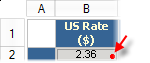To indicate cell referencing, PagePlus shows a colored indicator in the bottom-right corner of the cell being referenced. A different color is allocated to each cell reference made within the same formula.

Absolute cell references are set by using a dollar sign (“\$”) before the column or row cell reference (e.g., \$B\$2, \$B2, or B\$2). The dollar sign indicates that the reference will not change, even if the formula is copied and pasted, i.e.

• \$B\$2 – column and row reference are absolute (fixed) to cell B2.

• \$B2 – column reference is absolute to column B, but row reference is relative.

• B\$2 – column reference is relative, but row reference is absolute to row 2.

### Formula errors

When PagePlus can not understand a given formula, it returns an error in the cell containing the formula.

 #DIV0! Divide by Zero Error. Your calculation involves a divide by zero, e.g. A1/0, or A1/B1 if B1 has no number. #VALUE! Value Error. You have used a value which PagePlus was not expecting, e.g. providing a range of cells when a single cell is expected or vice-versa, or =A1:A2 instead of =SUM(A1:A2). #CIRC! Circular Reference Error. This cell refers to another cell which refers back to this one, in a loop. #SYNTAX! Syntax Error. You have entered a formula that PagePlus cannot interpret, e.g. =/-. #NAME! Name Error. You have used a function name that PagePlus does not know, e.g. =FRED(1). #REF! Reference Error. You have referenced a cell that does not exist. Usually a result of dragging or copying cells with relative references. #N/A Not Available Error. PagePlus does not have an available number. Usually because the formula refers to cell which contains an error.